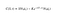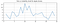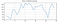# The coveted Black-Scholes model

This post details a model that has been prevalent since the 70s, developed by Fischer Black, Robert Merton, and Myron Scholes and is still widely used today. It is regarded as one of the best ways of determining fair prices of options. The underlying hypothesis is: By placing a certain set of assumptions on the market, the future price of the Options stock can be determined by modeling the movement of stock prices at different time intervals.

The Black–Scholes model is a mathematical model for pricing a derivative contract by simulating the dynamics of a financial market containing derivative financial instruments. The key property of the model is that it shows that an option has a unique price regardless of the risk of the underlying security and its expected return.

You can refer to my previous post to get an overview of financial derivative instruments. Before diving into the details of the Black-Scholes model, we’ll first cover Stochastic processes in brief as the Black-Scholes model formula is derived from a partial differential equation of a stochastic process.

# I. Stochastic processes:

A stochastic process means that one has a system for which there are observations at certain times and that the outcome, that is, the observed value at each time is a random variable. Simply put, at each observation at a certain time, there is a certain probability to get a certain outcome.

The stochastic evolution process is about using the probability distribution of a variable to evolve it over time.

Definition 1 : Mathematically, it is defined as a family of random variables, { X(t): t ∈ T}, where t usually denotes time. That is, at every time, t, in the set T, a random number X(t) is observed.

Example: Stochastic process with Bernoulli process:

It is a sequence of random variables, X1, X2, X3, varying with time. Where, X = [0,1] as it’s a Bernoulli trail. So, an event of sequence at time t1 can look like = [1,0,0,1,1,0].An event of sequence at time t1 can look like = [0,0,1,1,1,1]. Sample space here is a set of infinite sequences of 0s and 1s.

Definition 2: A stochastic process is a collection or ensemble of random variables indexed by a variable t, usually representing time. Let V(t) correspond to a collection of random variables for each time point t. This may be made explicit by specifying an event space Ω for the ensemble and for each event 𝜔∈Ω writing X(t, 𝜔) for the value taken by X at time t given the event 𝜔.

Thus, when the event 𝜔 is fixed and t is varied X(t, 𝜔) is a random function. Conversely, at each fixed time point t, X(t, 𝜔) spans the range of all possible values of X as the event 𝜔∈Ω varies and is thus a random variable.

Note that,

• X(t,ω) (or simply X(t)) is a random process.
• X(t0,ω) is a random variable for fixed t0.
• X(t,ω0) is a real-valued function of t for fixed ω0.
• X(t0,ω0) is a real number for fixed t0 and ω0.

A stochastic model is a tool for estimating probability distributions of potential outcomes by allowing for random variation in one or more inputs over time. The random variation is usually based on fluctuations observed in historical data for a selected period using standard time-series techniques.

A stochastic differential equation (SDE) is a differential equation in which one or more of the terms is a stochastic process, resulting in a solution which is also a stochastic process. To set up the premise, the derivation of the Black-Scholes formula starts with the modeling of a stochastic differential equation of the geometric Brownian motion(GBM). Log returns of the Options pricing are assumed to follow this motion.

The video gives a good description of Stochastic processes.

# II. Back to Black-Scholes Model:

The purpose of the model is to determine the price of a vanilla European call and put options (Option that can only be exercised at the end of its maturity) based on price variation over time and assuming the asset has a lognormal distribution. The model is based on a partial differential equation (PDE) which is modeling the behavior of geometric Brownian motion.

The original Black-Scholes model is based on a core assumption that the market consists of at least one risky asset (such as stock) and one (essentially) risk-free asset, such as a money market fund, cash, or a government bond. Additionally, it assumes three properties of the two assets, and four of the market itself:

Assumptions about the assets in the market:

1. The instantaneous log return of the risky asset’s price is assumed to behave as an infinitesimal random walk with constant drift and volatility, more precisely, according to geometric Brownian motion. This is to measure the volatility of the stock, which is calculated using the standard deviation of the log returns. The more volatile it is, the more valuable the option would be on that. Drift rate is the change in mean per unit of time.
2. The risky asset does not pay a dividend and follows a lognormal distribution of prices as stock prices can’t be negative.
3. The rate of return on the risk-free asset is constant (thus effectively behaves like an interest rate). This means that for a regular case if you were to purchase 100 shares, each worth of \$100, you’d need \$10,000. This is under the assumption that a trader borrows money for trading, which will lead to interest payments on this capital. Purchasing the call option at \$12 in a lot of 100 contracts will cost only \$1,200. Yet the profit potential will remain the same as that with a long stock position. Effectively, the differential of \$8,800 will result in savings of outgoing interest payment on this loaned amount. Alternatively, the saved capital of \$8,800 can be kept in an interest-bearing account and will result in interest income — a 5% interest will generate \$440 in one year.

1. There are no arbitrage (risk-free profit) opportunities.
2. It is possible to borrow and lend any amount of cash at the same rate as the interest rate of the risk-free asset.
3. It is possible to buy and sell any amount of the stock (including short selling).
4. There are no transaction costs in the market (i.e. no commission for buying or selling securities or derivative instruments).

# III. Linking the two together:

A stochastic Differential equation is used to model the distribution of Options pricing. The derivation of the Black-Scholes formula starts with the modeling of a stochastic differential equation of the geometric Brownian motion(GBM). Option pricing follows the GBM as explained above and here.

The instantaneous log return of the risky asset’s price is assumed to behave as an infinitesimal random walk with constant drift and volatility, more precisely, according to geometric Brownian motion.

A stochastic process St is said to follow a GBM if it satisfies the following stochastic differential equation (SDE):

where W_{t} is a Wiener process or Brownian motion, and 𝜇 (‘the percentage drift’) and 𝜎 (‘the percentage volatility’) are constants. A heuristic (but very helpful) interpretation of the stochastic differential equation is that in a small time interval of length δ the stochastic process St changes its value by an amount that is normally distributed with expectation μ(St, t) δ and variance σ(Square(St, t)) δ and is independent of the past behavior of the process. This is so because the increments of a Wiener process are independent and normally distributed. The function μ is referred to as the drift coefficient, while σ is called the diffusion coefficient. The stochastic process St is called a diffusion process and satisfies the Markov property.

The derivation of the Black-Scholes formula from the above-defined differential equation can be found on the wiki page. The final equation is provided in the section below:

C(S,t) is the cost price of the stock option at the time, t. S refers to the spot price of the asset at the time, t. T is the maturity of the option. Time to maturity is defined as T-t. K is the strike price of the option. r, the risk-free interest rate, assumed to be constant between t and T.Equation 2: C(S,t) is the value at time tt of a call option

where:

𝜎 refers to the volatility of the underlying asset, the standard deviation of the asset returns.

Strike price (also called exercise price) is the price at which you can buy the underlying security when exercising a call option or the price at which you can sell the underlying when exercising a put option. Spot price means the current market price

The first part, S*N(d1) is what you get ie the underlying stock if you decide to exercise your right to buy the stock.

The second part, K*e(-rt)*N(d2) is what you have to pay to receive that option. Thus the exercise price, i.e. K is multiplied by the discounting factor e(-rt) as this is the amount which we could have invested in a riskless asset instead of buying the option.

# IV. Experiments/ Results:

I ran a basic Black-Scholes model using the above-defined equation and have plotted Apple’s shares in the past.

• In multiple iterations of its run, the interest rate of the risk-free asset is constant along with the strike price and spot prices.
• Varying volatility
• The x-axis represents the time in weeks

Run-1: Call option prices for high volatility of Apple sharesA. Variation of the volatility of Apple shares against time (highly volatile). The volatility data is scraped from a publicly available source.B. Call options price variation with high volatility

Run-2: Call option prices for low volatility of Apple shares

As evident from the figures, high volatility results in more fluctuations in call prices, which leads to better profit margins.